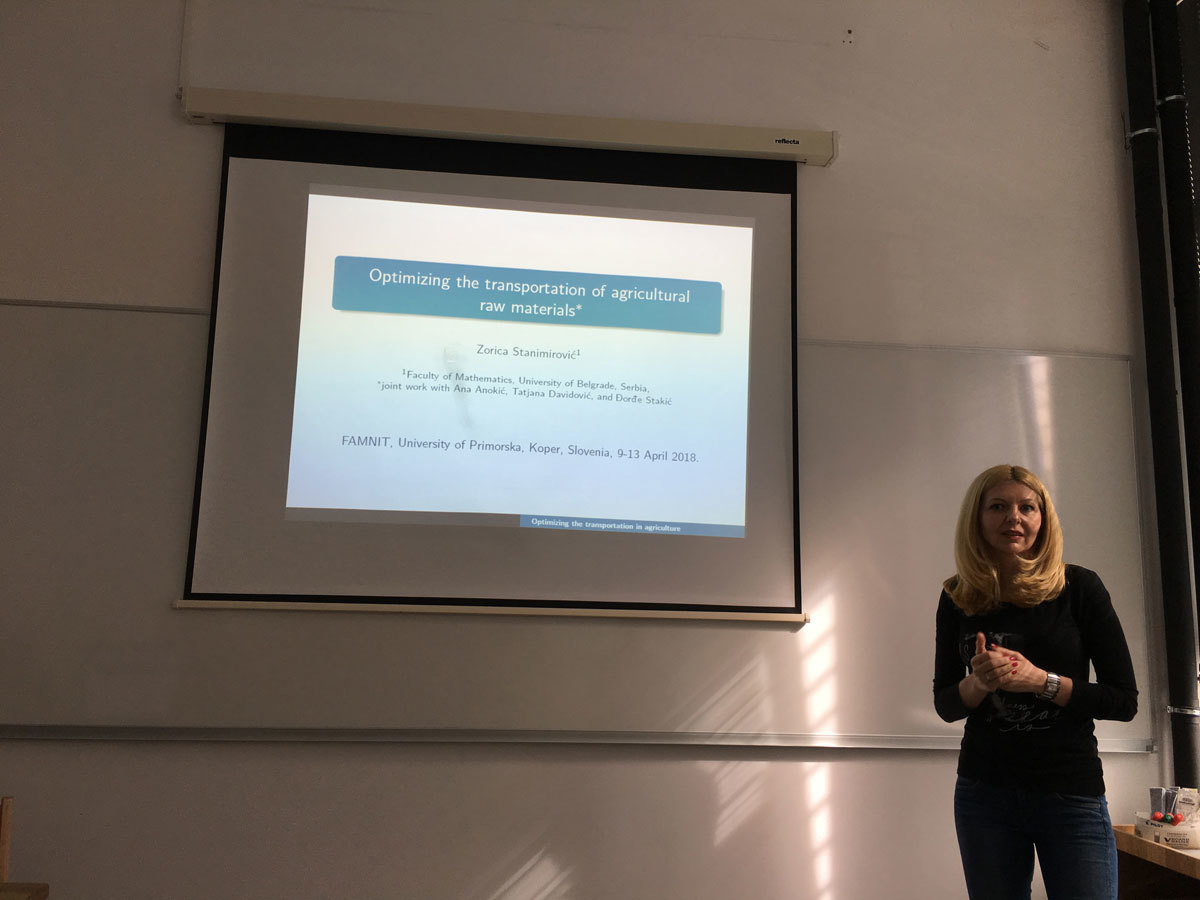Univerza na Primorskem Fakulteta za matematiko, naravoslovje in informacijske tehnologije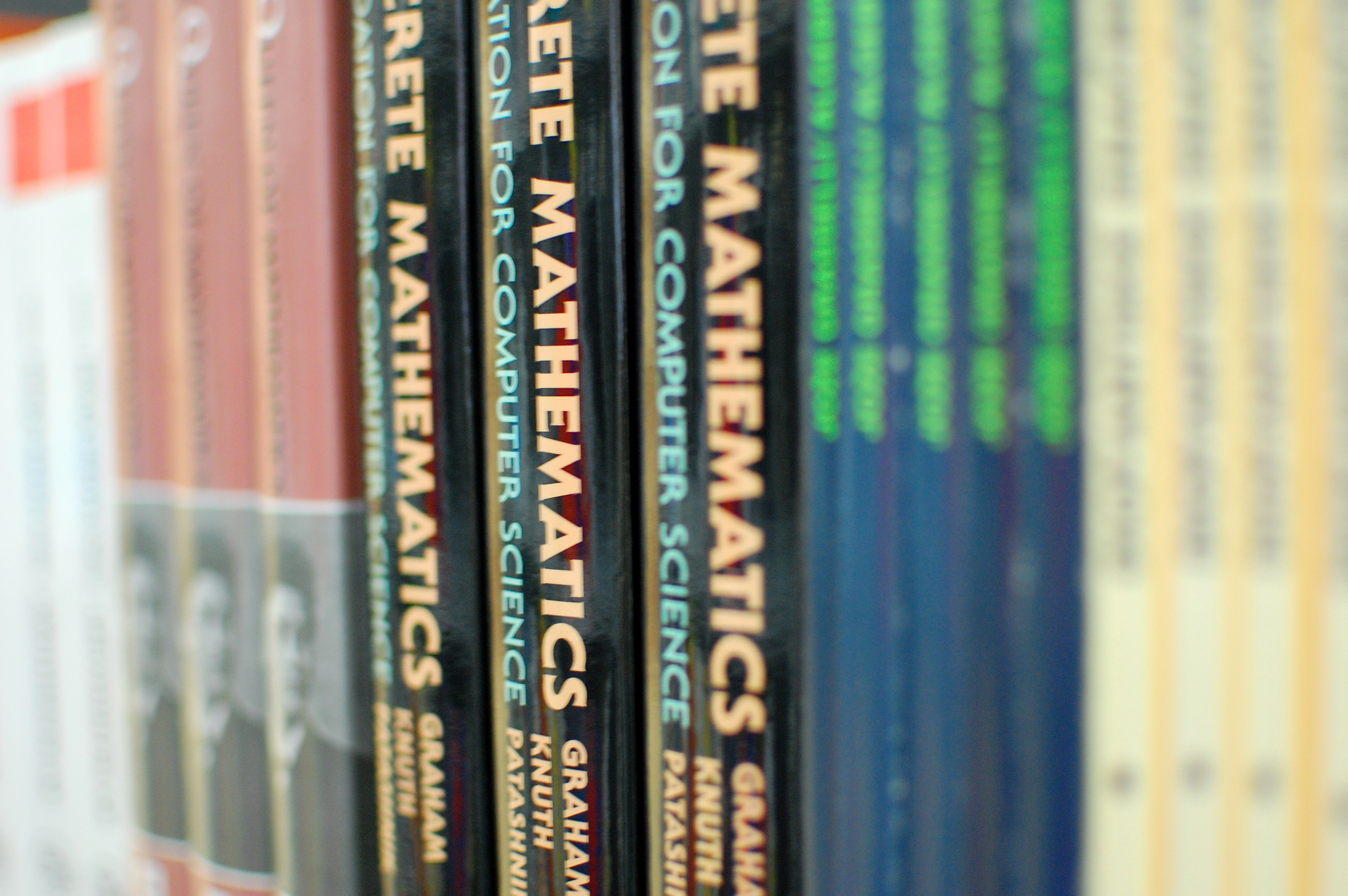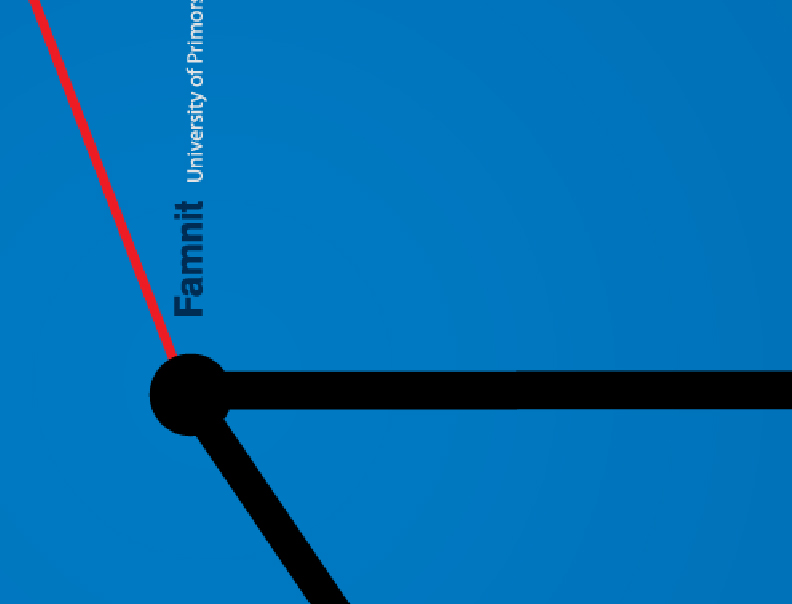SI | EN# Raziskovalni matematični seminar - Arhiv

 2021 2020 2019 2018 2017 2016 2015 2014 2013 2012 2011 2010
 1 2 3 4 5 6 7 8 9 10 11 12
Datum in ura / Date and time: 26.4.18
(10:00-11:00)
Predavalnica / Location: FAMNIT-MP1
Predavatelj / Lecturer: Peter Horak (University of Washington, Tacoma, USA)
Naslov / Title: Graph Theory as an Integral Part of Mathematics
Vsebina / Abstract:

Nowadays nobody doubts the significance of the applications of graph theory to other sciences or to real world problems. This workshop emphasizes the diversity of these applications.

However, not everyone outside of graph theory realizes that the powerful combinatorial methods found in graph theory have also been used to prove significant and well-known results in a variety of areas of pure mathematics. That is, that there are applications of graph theory to other areas of pure mathematics as well. Perhaps the best known of these methods are related to matching theory. For example, results from this area can be used to prove Dilworth's chain decomposition theorem for finite partially ordered sets. A well-known application of matching in group theory shows that there is a common set of left and right coset representatives of a subgroup in a finite group. Also, the existence of matchings in certain infinite bipartite graphs played an important role in Laczkovich's affirmative answer to Tarski's 1925 problem of whether a circle is piecewise congruent to a square . Other applications of graph theory to pure mathematics may be found scattered throughout the literature, e.g. see . There, among other examples, matching theory is applied to give a very simple constructive proof of the existence of Haar measure on compact topological groups.

In this talk we will present an examples of such applications of graph theory to number theory, measure theory, and analysis, respectively. On purpose two of these applications are applications of graph theory (finite mathematics) to continuous mathematics, which we find more impressive. Further criteria for choosing these examples were that the theorem is fundamental, widely known, and the graph theory proof of the theorem is the most elegant and/or the shortest one. In other words, the proof should exhibit the strength and elegance of graph-theoretic methods.

References
 M. Laczkovich, M. Equidecomposability and discrepancy; a solution of Tarski's circle-squaring problem. J. Reine Angew. Math. 404 (1990), 77--117.
 L. Lóvasz, L. Pyber, D. J. A.Welsh, and G. M. Ziegler, Combinatircs in pure mathematics, in Handbook of Combinatorics (R.L. Graham, M. Grotschel, and L. Lovasz, eds.), Elsevier, 1996.

Datum in ura / Date and time: 23.4.18
(10:00-11:00)
Predavalnica / Location: FAMNIT-POŠTA
Predavatelj / Lecturer: Micael Toledo (UP FAMNIT)
Naslov / Title: On cubic vertex-transitive tricirculants
Vsebina / Abstract:

For a positive integer k, we say a simple graph G is a k-circulant if it admits a semiregular automorphism that partitions its vertex-set into k orbits of the same size. The study of k-circulant graphs is extensive, specially that of vertex- and arc-transitive ones, and some families of k-circulants, for small values of k, are of particular interest. We provide a complete classification, along with some structural results, for cubic vertex-transitive 3-circulants.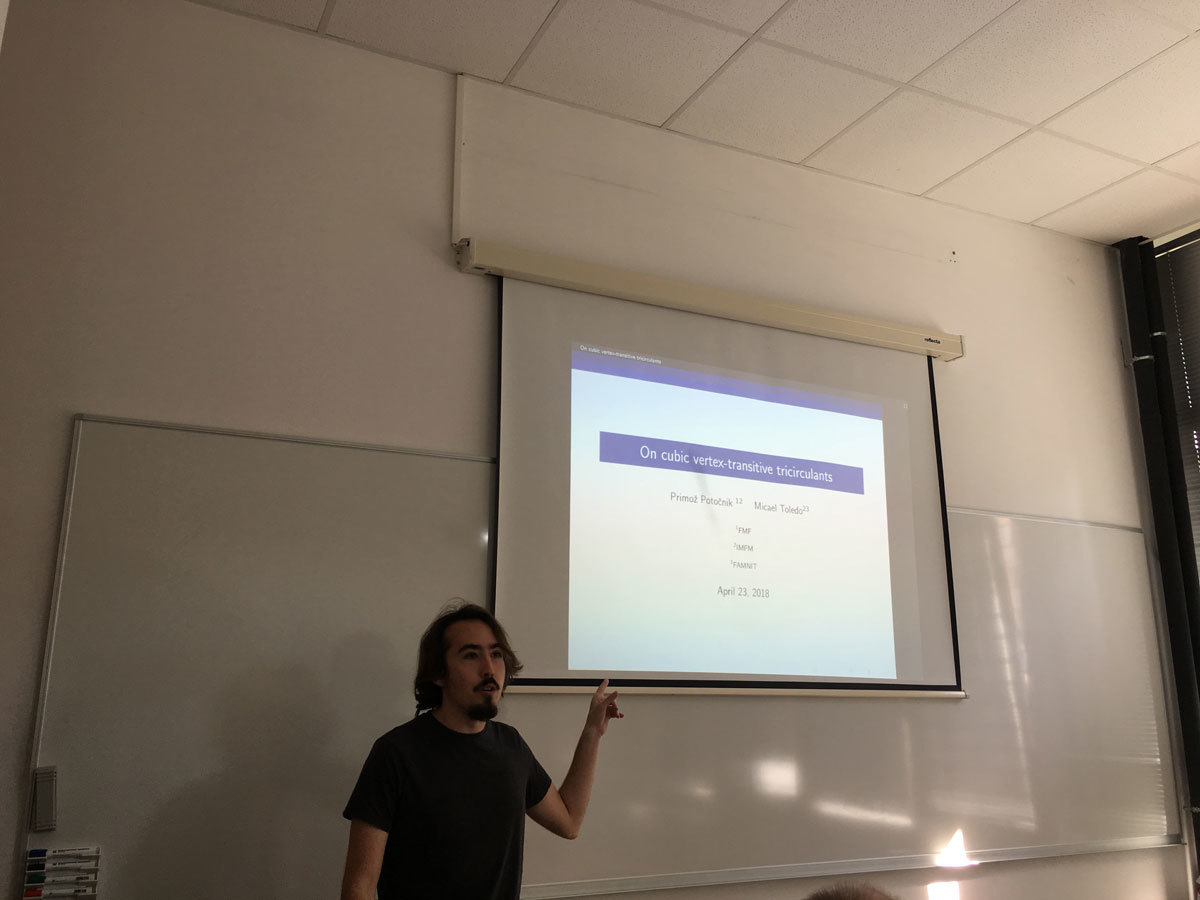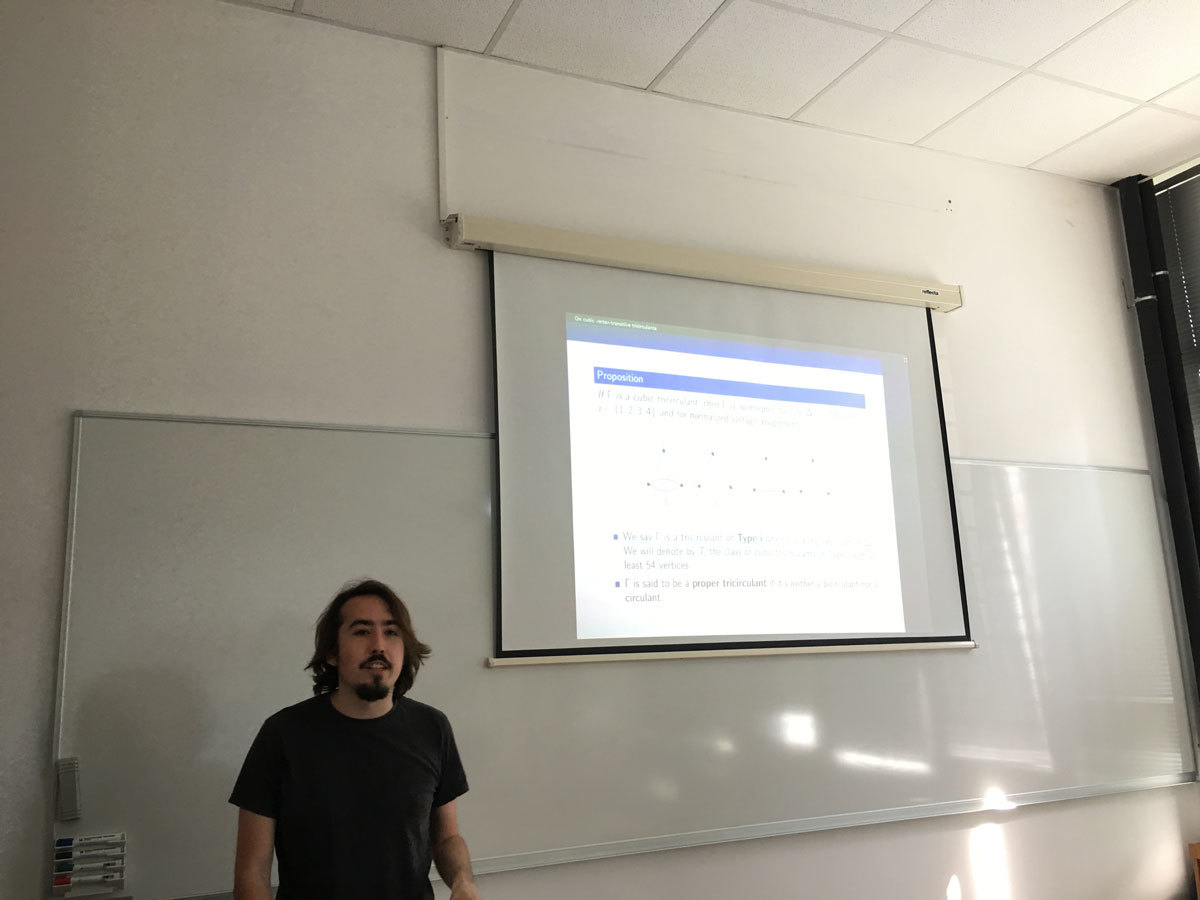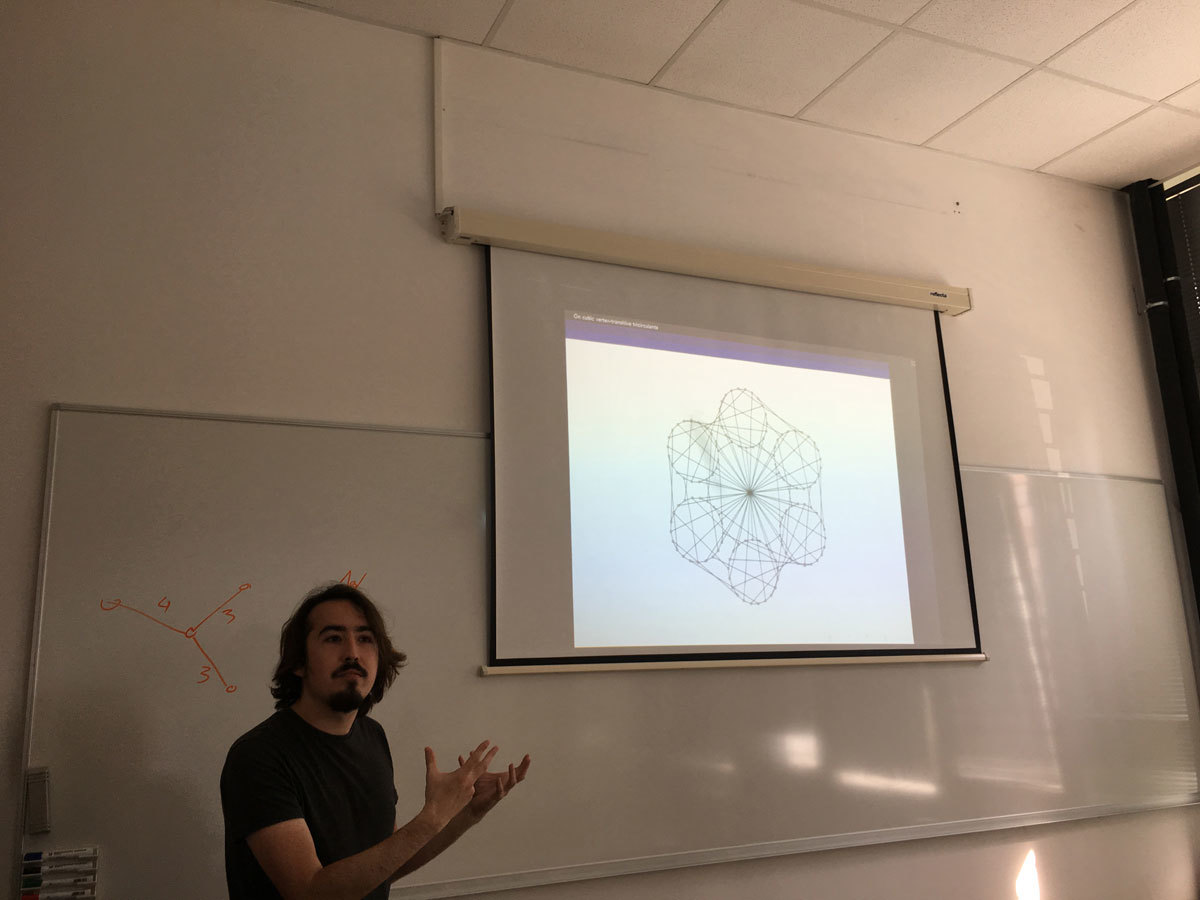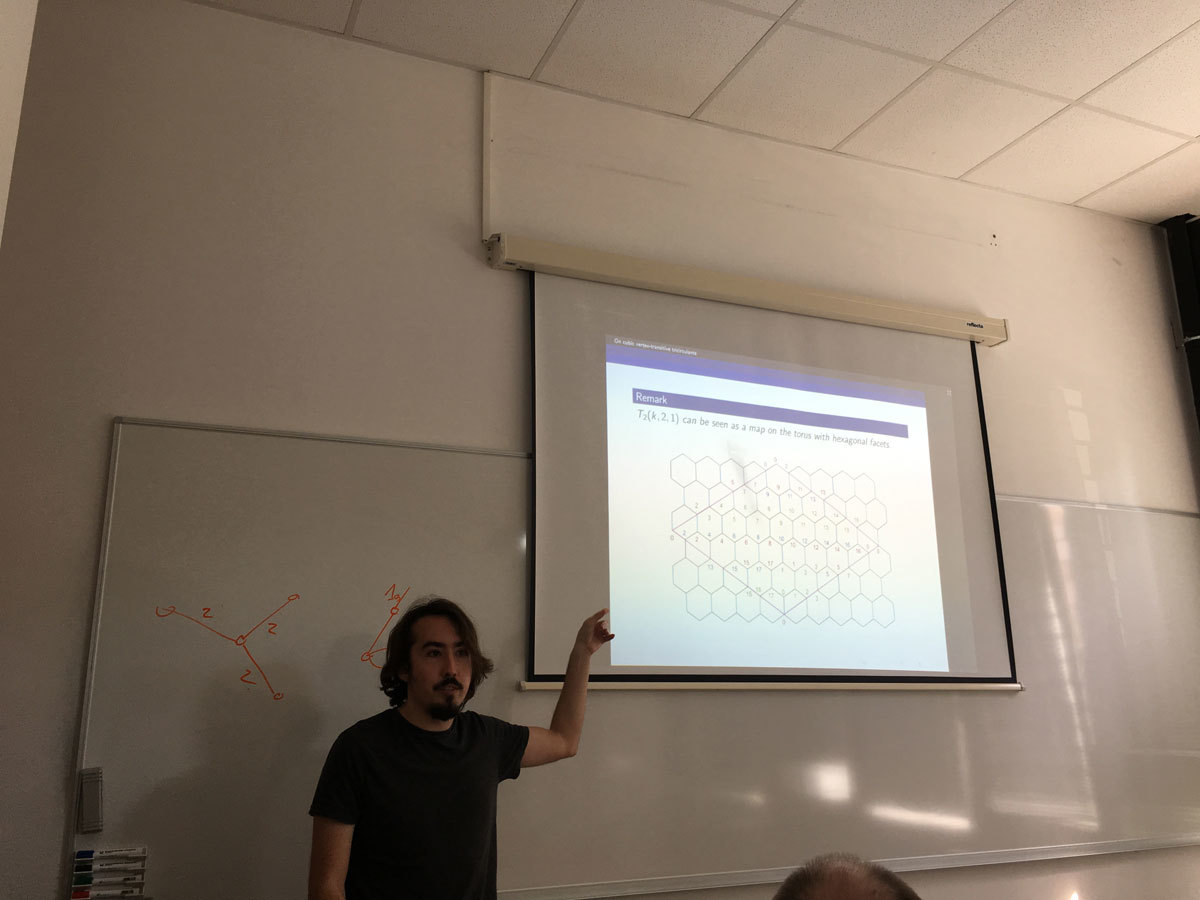Datum in ura / Date and time: 16.4.18
(10:00-11:00)
Predavalnica / Location: FAMNIT-POŠTA
Predavatelj / Lecturer: Sylwia Cichacz (AGH University of Science and Technology in Kraków, Poland)
Naslov / Title: The zero sum partition of Abelian groups and its applications
Vsebina / Abstract:

Kaplan, Lev and  Roditty  introduced a notion of zero-sum partitions of subsets in Abelian groups. Let \Gamma be an Abelian group of order n. We shall say that \Gamma has the zero-sum-partition property (ZSP-property) if every partition n-1 = r_1 + r_2 + \ldots + r_t of n-1, with r_i \geq 2 for 1 \leq i \leq t and for any possible positive integer t, there is a partition of \Gamma - \{0\} into pairwise disjoint subsets A_1, A_2,\ldots , A_t, such that |A_i| = r_i and \sum_{a\in A_i}a = 0 for 1 \leq i \leq t.  They conjectured that every Abelian group \Gamma, which is of odd order or contains exactly three involutions, has the ZSP-property. The conjecture was recently proved by Zeng .

In this talk we show some applications of the ZSP-property of groups in some graph labeling problems.

 G. Kaplan, A. Lev, Y. Roditty, On zero-sum partitions and anti-magic trees, Discrete Math. 309(8) (2009), 2010--2014.
 X. Zeng, On zero-sum partitions of abelian groups. Integers 15 (2015), Paper No. A44, 16 pp.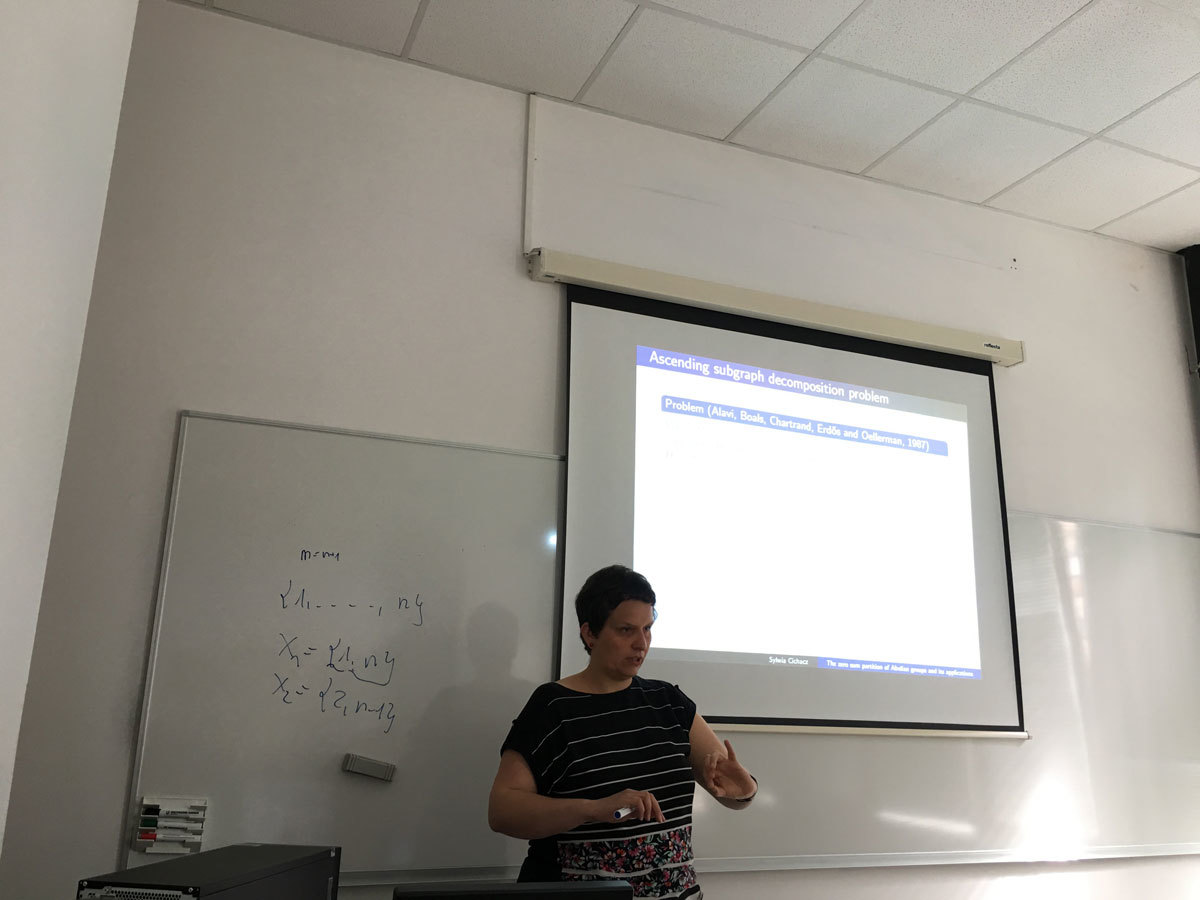Datum in ura / Date and time: 9.4.18
(10:00-11:00)
Predavalnica / Location: FAMNIT-POŠTA
Predavatelj / Lecturer: Zorica Stanimirović (Faculty of Mathematics, University of Belgrade)
Naslov / Title: A vehicle scheduling problem in optimizing the transportation of agriculture raw materials
Vsebina / Abstract:

A variant of Vehicle Scheduling Problem (VSP) arising from the sugar beet transportation in a sugar factory in Serbia is introduced. The objective of the considered VSP is to minimize the required transportation time under problem-specific constraints. The considered VSP is first modelled as Mixed Integer Quadratically Constrained Program (MIQCP) and then reformulated to the equivalentMixed Integer Linear Program (MILP). In addition, it is proved that the considered VSP is NP-hard. Both MIQCP and MILP formulations were used within the framework of Lingo 17 solver on the set of real-life and generated problem instances. The proposed formulations enable Lingo 17 solver to produce optimal or feasible solutions for smaller-size problem dimensions. On these test examples, MILP reformulation showed to be superior over the MIQCP model in terms of solution quality and running times required by Lingo 17 solver.

This is a joint work with Anokić Ana, Tatjana Davidović and Đorđe Stakić.